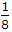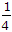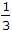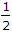# Civil Engineering - Strength of Materials - Discussion

### Discussion :: Strength of Materials - Section 1 (Q.No.2)

2.

As compared to uniaxial tension or compression, the strain energy stored in bending is only

 [A].[B].[C].[D].Explanation:

No answer description available for this question.

 Samrat said: (Oct 11, 2014) How it is calculated?

 Dipankar said: (Oct 29, 2014) Due to middle-third rule.

 Subinsekhar said: (Nov 3, 2014) Can you explain?

 Syed Aasif said: (Feb 25, 2015) We know strain energy stored is direct stress square/2.

 Akash said: (Jul 9, 2015) Step 1: Calculate strain energy due to uniaxial loading. Viz. U1 = {(f^2)xvol}/(2xE). Step 2: Calculate strain energy due to pure bending. Viz. U2 = 0.5xMxtheta. = 0.5x[(fxI)/y]x[(Mxl)/(ExI)]. (from simple bending equation & moment area method resp.) Solve it U2 = {(f^2)xvol}/(6xE). i.e U2 = 0.3XU1. :) :) :) :).

 Subhra said: (Oct 9, 2015) @Akash, Please explain me what is l?

 Binit said: (Nov 13, 2015) Need more explanation.

 Dheeraj Hindu said: (Aug 9, 2016) We know that, FOR COMPRESSIOM OR TENSIOIN. Strain energy(SE) = (1/2) * force * deformation = 1/2) * P * PL/AE = P^2L/2AE. FOR BENDING. SE = (P^2L^3)/6AE. So bending (SE)= (L^2)/3 tension(SE).

 Naren said: (Jan 14, 2017) Here, [SE] FOR COMPRESSION OR TENSION IS. = 1/2 * force * deformation. And what about [SE] for BENDING. @Dheeraj Hindu. Could you please explain this one.

 Srinivas said: (Feb 25, 2017) How it is calculated? Please explain me.

 Surya said: (Apr 5, 2017) Please give the explanation.

 Hiralal Singha said: (Jun 13, 2017) Not getting this, Explain it please.

 Deepak said: (Jul 22, 2017) How to solve this? please explain.

 Maneesha.Puvvala said: (Aug 15, 2017) Can you give me a deep explanation?

 Ajay Munde said: (Oct 28, 2017) Not getting this. Please explain.

 Shakil said: (Jan 7, 2018) Due to avail stress =σ2/2E.vol. Due bending = Σ2/6E.vol.

 Jeldi said: (Jan 29, 2018) U= (σ^2*v)/2E -----> (axial loading) U=(σ^2*v)/6E -----> (bending) So ans is (1/3) For bending U=Ʃ[ (M^2)/2E ] dx{hear integration frm 0 to L} U=[ (M^2)/2E ]*Ʃ1dx U=[ (M^2)/2E ]*L Now M/I = E/R=σ/y So M=σ*I/y On simplifying U=(σ^2*v)/6E

 Shalu said: (Mar 6, 2018) Can't understand can you explain?

 Shiney said: (Mar 30, 2018) Will you please explain it clearly?

 Sarang Mote said: (Jan 21, 2019) But the strain energy if load multiplied by displacement, Then in case of bending, how do we derive the formula.

 Pawan said: (Mar 21, 2019) U= (σ^2*v)/2E -----> (axial loading). U=(σ^2*v)/6E -----> (bending). So, answer is (1/3).

 Abhishek Thakur said: (Jul 11, 2019) For(tension and compression): Due to the stress-strain curve the strain energy; Resilience = 1/2 * stress * strain. And proof resilience= 1/2* stress * strain * volume is; Strain = stress/modulus of elasticity. Then PR=stress^2*volume/2E. And for bending moment due to pure axial loading. M=Wx. Strain energy due to bending moment = integration of M^2*xdx/2E= integration of (Wx)^2dx/2E (limit 0toL). Put the value of Moment M here and on solving u will get; W^2 l^3/6E. On solving both the value you will get; 1/3.

 Fahad said: (Oct 7, 2020) Thanks @Abhishek Thakur.